Log

if, what is b?

Result

b =  2.3

Solution:Leave us a comment of example and its solution (i.e. if it is still somewhat unclear...):Be the first to comment!Next similar examples:

1. Computer revolution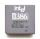When we started playing with computers, the first processor, which I remember was the Intel 8080 from 1974, with the performance of 0.5 MIPS. Calculate how much percent a year rose CPU performance when Intel 486DX from 1992 has 54 MIPS. What
2. SequenceCalculate what member of the sequence specified by ? has value 86.
3. DemographicsThe population grew in the city in 10 years from 42000 to 54500. What is the average annual percentage increase of population?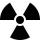After 548 hours decreases the activity of a radioactive substance to 1/9 of the initial value. What is the half-life of the substance?
5. Powers 3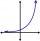2 to the power of n divided by 4 to the power of -3 equal 4. What is the vaule of n?
6. Intercept with axisF(x)=log(x+4)-2, what is the x intercept
7. Virus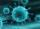We have a virus that lives one hour. Every half hour produce two child viruses. What will be the living population of the virus after 3.5 hours?
8. Exponential equationIn the set R solve the equation: ?
9. The city 3The city has 22,000 residents. How long it is expected to have 25,000 residents if the average annual population growth is 1.4%?
10. Coordinate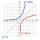Determine missing coordinate of the point M [x, 120] of the graph of the function f bv rule: y = 5x
11. Geometric progressionIn geometric progression, a1 = 7, q = 5. Find the condition for n to sum first n members is: sn≤217.
12. Exponential equationSolve for x: (4^x):0,5=2/64.
13. Car valueThe car loses value 15% every year. Determine a time (in years) when the price will be halved.
14. Exponential equationSolve exponential equation (in real numbers): 98x-2=9
15. LogarithmDetermine the number whose decimal logarithm is -3.8.
16. Theorem proveWe want to prove the sentence: If the natural number n is divisible by six, then n is divisible by three. From what assumption we started?
17. Annual pensionCalculate the amount of money generating an annual pension of EUR 1000, payable at the end of the year and for a period of 10 years, shall be inserted into the bank to account with an annual interest rate of 2%